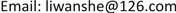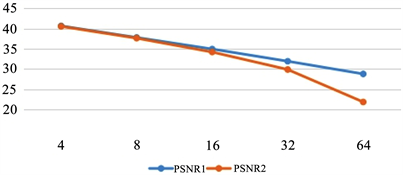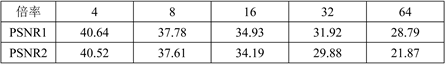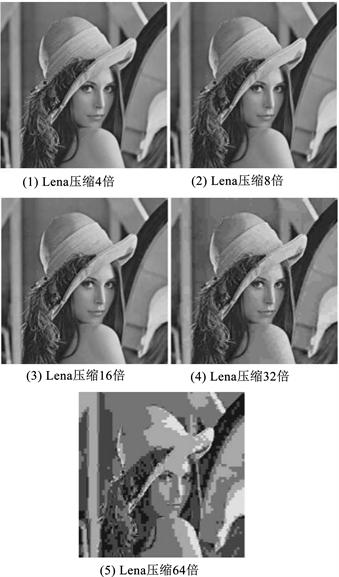﻿ 基于双密度双树复小波变换的图像压缩 Image Compression Based on Dual Density Dual Tree Complex Wavelet Transform

Journal of Image and Signal Processing
Vol. 08  No. 01 ( 2019 ), Article ID: 28107 , 6 pages
10.12677/JISP.2019.81002

Image Compression Based on Dual Density Dual Tree Complex Wavelet Transform

Wanshe Li, Ruizhi Zhu

School of Mathematics and Information Science, Shaanxi Normal University, Xi’an ShaanxiReceived: Nov. 27th, 2018; accepted: Dec. 13th, 2018; published: Dec. 20th, 2018ABSTRACT

With the rapid development of multimedia information technology, the extremely large image data is produced. When these massive image data are stored and transmitted, it is necessary to use image compression coding technology to reduce the amount of data. The purpose of this study is to find a method of a better quality of compressed image. The paper is based on the theory of wavelet transform and image compression, and an image compression method based on dual-density dual-tree complex wavelet transform is proposed. According to MATLAB simulation, experimental results are given. Finally, it is confirmed that this method has obvious optimization compared with the traditional image compression method for image compression quality.

Keywords:Wavelet Transform, Image Compression, Double-Density Dual-Tree Complex Wavelet TransformCopyright © 2019 by authors and Hans Publishers Inc.1. 引言

2. 双密度双树复小波变换

2.1. 双树复小波变换

$\psi \left(t\right)={\psi }_{h}\left(t\right)+j{\psi }_{g}\left(t\right)$ (2-1)

${\psi }_{h}\left(t\right)\approx H\left\{{\psi }_{g}\left(t\right)\right\}$ (2-2)

$\psi \left(x,y\right)=\psi \left(x\right)\psi \left(y\right)$ ，由(2-1)代入可得

$\begin{array}{c}\psi \left(x,y\right)=\left[\left({\psi }_{h}\left(x\right)+j{\psi }_{g}\left(x\right)\right)\right]\left[\left({\psi }_{h}\left(y\right)+j{\psi }_{g}\left(y\right)\right)\right]\\ ={\psi }_{h}\left(x\right){\psi }_{h}\left(y\right)-{\psi }_{g}\left(x\right){\psi }_{g}\left(y\right)+j\left[{\psi }_{g}\left(x\right){\psi }_{h}\left(y\right)+{\psi }_{h}\left(x\right){\psi }_{g}\left(y\right)\right]\end{array}$

2.2. 双密度小波变换

$\left\{\begin{array}{l}\phi \left(t\right)=\sqrt{2}\underset{n}{\sum }{h}_{0}\left(n\right)\phi \left(2t-n\right)\\ {\psi }_{1}\left(t\right)=\sqrt{2}\underset{n}{\sum }{h}_{1}\left(n\right)\phi \left(2t-n\right)\\ {\psi }_{2}\left(t\right)=\sqrt{2}\underset{n}{\sum }{h}_{2}\left(n\right)\phi \left(2t-n\right)\end{array}$ (2-3)

2.3. 双密度双树复小波变换的获取

$\left\{\begin{array}{c}{\psi }_{h,1}\left(t\right)={\psi }_{h,2}\left(t-0.5\right)\\ {\psi }_{g,1}\left(t\right)={\psi }_{g,2}\left(t-0.5\right)\end{array}$ (2-4)

$\left\{\begin{array}{c}{\psi }_{g,1}\left(t\right)=H\left({\psi }_{h,1}\left(t\right)\right)\\ {\psi }_{g,2}\left(t\right)=H\left({\psi }_{h,2}\left(t\right)\right)\end{array}$ (2-5)

$\varphi \left(t\right)={\varphi }_{h}\left(t\right)+j{\varphi }_{g}\left(t\right)$ (2-6)

$\left\{\begin{array}{c}{\psi }_{1}\left(t\right)={\psi }_{h,1}\left(t\right)+j{\psi }_{g,1}\left(t\right)\\ {\psi }_{2}\left(t\right)={\psi }_{h,2}\left(t\right)+j{\psi }_{g,2}\left(t\right)\end{array}$ (2-7)

3. 基于双密度双树复小波变换的图像压缩

3.1. 图像质量评价标准

$\text{PSNR}=10\mathrm{log}\left(\frac{{255}^{2}}{MSE}\right)$ (3-1)

$MSE=\frac{1}{w×h}\underset{i=0}{\overset{w-1}{\sum }}\underset{i=0}{\overset{h-1}{\sum }}{\left(a\left[i\right]\left[j\right]-b\left[i\right]\left[j\right]\right)}^{2}$ (3-2)

3.2. 实验结果比较和分析Figure 1. PSNR value comparison diagram of the two compression methodsFigure 2. Compression Lena graph based on dual density dual tree complex wavelet transformFigure 3. JPEG standard compressed Lena

4. 总结

Image Compression Based on Dual Density Dual Tree Complex Wavelet Transform[J]. 图像与信号处理, 2019, 08(01): 9-14. https://doi.org/10.12677/JISP.2019.81002

1. 1. 张春田, 苏育挺, 张静. 数字图像压缩编码[M]. 北京: 清华大学出版社, 2006.

2. 2. 伯勒斯. 小波与小波变换导论[M]. 北京: 电子工业出版社, 2013.

3. 3. Wu, Y.J., Deng, Y., Duan, H.B. and Zhou, L.N. (2014) Dual Tree Complex Wavelet Transform Ap-proach to Copy-Rotate-Move Forgery Detection. Science China (Information Sciences), 57, 125-136.

4. 4. Jung, C., Yang, Q., Sun, T.T., Fu, Q.T. and Song, H. (2017) Low Light Image Enhancement with Dual-Tree Complex Wavelet Transform. Journal of Visual Communication and Image Representation, 42.

5. 5. 王海旭, 吴绍华, 杨竞然, 丁婵娟. 基于双密度双树复小波的结构化CS图像重构[J]. 应用科学学报, 2016, 34(2): 115-126.

6. 6. 王高峰, 石钟磊, 谭魏盟, 李思, 高涛. 融合双密度双树复小波变换与多尺度Retinex的图像增强算法[J]. 计算机与数字工程, 2017, 45(5): 870-873+934.